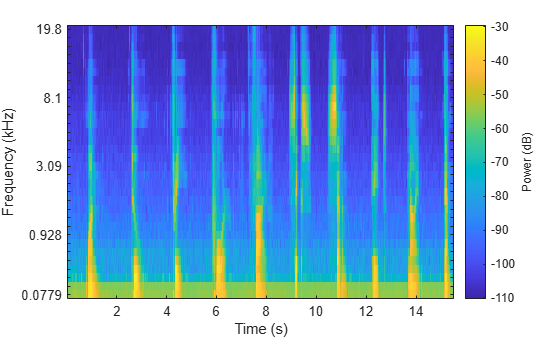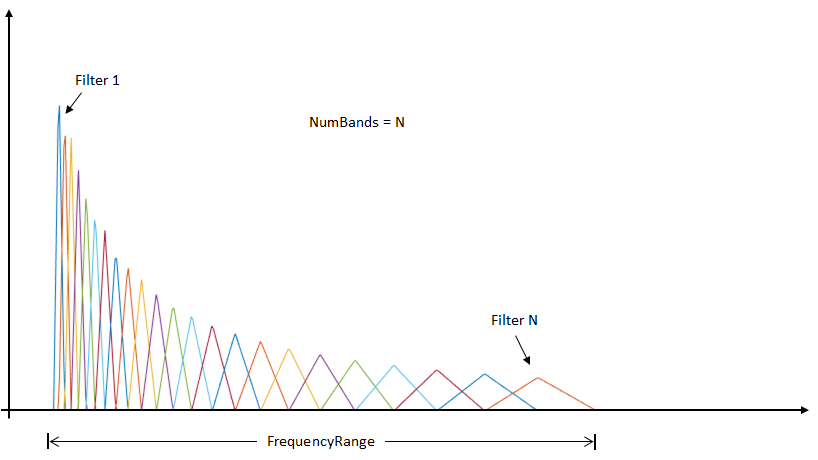# melSpectrogram

Mel spectrogram

## Syntax

``S = melSpectrogram(audioIn,fs)``
``S = melSpectrogram(audioIn,fs,Name,Value)``
``[S,F,T] = melSpectrogram(___)``
``melSpectrogram(___)``

## Description

example

````S = melSpectrogram(audioIn,fs)` returns the mel spectrogram of the audio input at sample rate `fs`. The function treats columns of the input as individual channels.```

example

````S = melSpectrogram(audioIn,fs,Name,Value)` specifies options using one or more `Name,Value` pair arguments.```

example

````[S,F,T] = melSpectrogram(___)` returns the center frequencies of the bands in Hz and the location of each window of data in seconds. The location corresponds to the center of each window. You can use this output syntax with any of the previous input syntaxes.```

example

````melSpectrogram(___)` plots the mel spectrogram on a surface in the current figure.```

## Examples

collapse all

Use the default settings to calculate the mel spectrogram for an entire audio file. Print the number of bandpass filters in the filter bank and the number of frames in the mel spectrogram.

```[audioIn,fs] = audioread('Counting-16-44p1-mono-15secs.wav'); S = melSpectrogram(audioIn,fs); [numBands,numFrames] = size(S); fprintf("Number of bandpass filters in filterbank: %d\n",numBands)```
```Number of bandpass filters in filterbank: 32 ```
`fprintf("Number of frames in spectrogram: %d\n",numFrames)`
```Number of frames in spectrogram: 1551 ```

Plot the mel spectrogram.

`melSpectrogram(audioIn,fs)`Calculate the mel spectrums of 2048-point periodic Hann windows with 1024-point overlap. Convert to the frequency domain using a 4096-point FFT. Pass the frequency-domain representation through 64 half-overlapped triangular bandpass filters that span the range 62.5 Hz to 8 kHz.

```[audioIn,fs] = audioread('FunkyDrums-44p1-stereo-25secs.mp3'); S = melSpectrogram(audioIn,fs, ... 'Window',hann(2048,'periodic'), ... 'OverlapLength',1024, ... 'FFTLength',4096, ... 'NumBands',64, ... 'FrequencyRange',[62.5,8e3]);```

Call `melSpectrogram` again, this time with no output arguments so that you can visualize the mel spectrogram. The input audio is a multichannel signal. If you call `melSpectrogram` with a multichannel input and with no output arguments, only the first channel is plotted.

```melSpectrogram(audioIn,fs, ... 'Window',hann(2048,'periodic'), ... 'OverlapLength',1024, ... 'FFTLength',4096, ... 'NumBands',64, ... 'FrequencyRange',[62.5,8e3])````melSpectrogram` applies a frequency-domain filter bank to audio signals that are windowed in time. You can get the center frequencies of the filters and the time instants corresponding to the analysis windows as the second and third output arguments from `melSpectrogram`.

Get the mel spectrogram, filter bank center frequencies, and analysis window time instants of a multichannel audio signal. Use the center frequencies and time instants to plot the mel spectrogram for each channel.

```[audioIn,fs] = audioread('AudioArray-16-16-4channels-20secs.wav'); [S,cF,t] = melSpectrogram(audioIn,fs); S = 10*log10(S+eps); % Convert to dB for plotting for i = 1:size(S,3) figure(i) surf(t,cF,S(:,:,i),'EdgeColor','none'); xlabel('Time (s)') ylabel('Frequency (Hz)') view([0,90]) title(sprintf('Channel %d',i)) axis([t(1) t(end) cF(1) cF(end)]) end```## Input Arguments

collapse all

Audio input, specified as a column vector or matrix. If specified as a matrix, the function treats columns as independent audio channels.

Data Types: `single` | `double`

Input sample rate in Hz, specified as a positive scalar.

Data Types: `single` | `double`

### Name-Value Arguments

Specify optional pairs of arguments as `Name1=Value1,...,NameN=ValueN`, where `Name` is the argument name and `Value` is the corresponding value. Name-value arguments must appear after other arguments, but the order of the pairs does not matter.

Before R2021a, use commas to separate each name and value, and enclose `Name` in quotes.

Example: `'WindowLength',1024`

Window applied in time domain, specified as the comma-separated pair consisting of `'Window'` and a real vector. The number of elements in the vector must be in the range [1,`size(audioIn,1)`]. The number of elements in the vector must also be greater than `OverlapLength`.

Data Types: `single` | `double`

Analysis window overlap length in samples, specified as the comma-separated pair consisting of `'OverlapLength'` and an integer in the range `[0, (WindowLength - 1)]`.

Data Types: `single` | `double`

Number of points used to calculate the DFT, specified as the comma-separated pair consisting of `'FFTLength'` and a positive integer greater than or equal to `WindowLength`. If unspecified, `FFTLength` defaults to `WindowLength`.

Data Types: `single` | `double`

Number of mel bandpass filters, specified as the comma-separated pair consisting of `'NumBands'` and a positive integer.

Data Types: `single` | `double`

Frequency range over which to compute the mel spectrogram in Hz, specified as the comma-separated pair consisting of `'FrequencyRange'` and a two-element row vector of monotonically increasing values in the range ```[0, fs/2]```.

Data Types: `single` | `double`

Type of mel spectrogram, specified as the comma-separated pair consisting of `'SpectrumType'` and `'power'` or `'magnitude'`.

Data Types: `char` | `string`

Apply window normalization, specified as the comma-separated pair consisting of `'WindowNormalization'` and `true` or `false`. When `WindowNormalization` is set to `true`, the power (or magnitude) in the mel spectrogram is normalized to remove the power (or magnitude) of the time domain `Window`.

Data Types: `char` | `string`

Type of filter bank normalization, specified as the comma-separated pair consisting of `'FilterBankNormalization'` and `'bandwidth'`, `'area'`, or `'none'`.

Data Types: `char` | `string`

## Output Arguments

collapse all

Mel spectrogram, returned as a column vector, matrix, or 3-D array. The dimensions of `S` are L-by-M-by-N, where:

• L is the number of frequency bins in each mel spectrum. `NumBands` and `fs` determine L.

• M is the number of frames the audio signal is partitioned into. `size(audioIn,1)`, `WindowLength`, and `OverlapLength` determine M.

• N is the number of channels such that N = `size(audioIn,2)`.

Trailing singleton dimensions are removed from the output `S`.

Data Types: `single` | `double`

Center frequencies of mel bandpass filters in Hz, returned as a row vector with length `size(S,1)`.

Data Types: `single` | `double`

Location of each analysis window of audio in seconds, returned as a row vector length `size(S,2)`. The location corresponds to the center of each window.

Data Types: `single` | `double`

## Algorithms

collapse all

The `melSpectrogram` function follows the general algorithm to compute a mel spectrogram as described in .In this algorithm, the audio input is first buffered into frames of `numel(Window)` number of samples. The frames are overlapped by `OverlapLength` number of samples. The specified `Window` is applied to each frame, and then the frame is converted to frequency-domain representation with `FFTLength` number of points. The frequency-domain representation can be either magnitude or power, specified by `SpectrumType`. If `WindowNormalization` is set to `true`, the spectrum is normalized by the window. Each frame of the frequency-domain representation passes through a mel filter bank. The spectral values output from the mel filter bank are summed, and then the channels are concatenated so that each frame is transformed to a `NumBands`-element column vector.

### Filter Bank Design

The mel filter bank is designed as half-overlapped triangular filters equally spaced on the mel scale. `NumBands` controls the number of mel bandpass filters. `FrequencyRange` controls the band edges of the first and last filters in the mel filter bank. `FilterBankNormalization` specifies the type of normalization applied to the individual bands.Rabiner, Lawrence R., and Ronald W. Schafer. Theory and Applications of Digital Speech Processing. Upper Saddle River, NJ: Pearson, 2010.